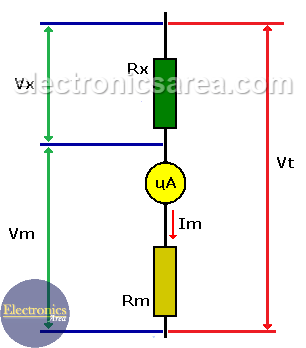# How to extend the voltage range of an analog Multimeter?

In order to extend the voltage range of an analog multimeter, a resistor is used. This resistor is placed in series with the multimeter, as seen in the figure below.

The voltage between the terminals of an analog voltmeter is 100mV, when the needle is at full scale.

The resistor Rx that is placed in series with the internal resistance of the voltmeter (Rm), creates a voltage divider.  The voltage in each of the series resistors is proportional to the values  that these resistors have. Then:  Vx / Vm = Rx / Rm.

The total resistance of the voltmeter is: Rt = Rx + Rm. From the last equation Rx is cleared and we obtain: Rx = Rt – Rm. Rt can be obtained by dividing the total voltage (Vt) by the current (Im): Rt = Vt / Im, and we get: Rx = (Vt/Im) – Rm.## Calculation example to extend the analog voltmeter range.

In this case the range of the instrument will be extended to measure voltage from 100mV to 1 Volt. The data we have are:

• Vt = 1V,
• Im = 50uA, and …
• Rm = 2K

Substituting these values in the previous formula: Rx = (Vt/Im) – Rm.

Rx = (1/(50 x 10-6)) – 2 x 103 = 18 x 103 = 18K. Then the necessary resistor value is 18K.

This type of resistors in series (to change the voltage range) and resistors in parallel / SHUNT (to change the current range), are those that typical analogue multimeters have in order to change the measurement scale.

• 1
•
•
•
•
•
•
•
•
1
Share
•
1
Share
• 1
•
•
•
•
•
•
•